# 2.5 Solve equations with fractions or decimals  (Page 2/2)

 Page 2 / 2

Solve: $-11=\frac{1}{2}\left(6p+2\right)$ .

$p=-4$

Solve: $8=\frac{1}{3}\left(9q+6\right)$ .

$q=2$

In the next example, even after distributing, we still have fractions to clear.

Solve: $\frac{1}{2}\left(y-5\right)=\frac{1}{4}\left(y-1\right)$ .

## Solution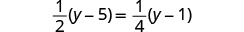Distribute.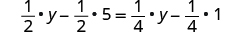Simplify.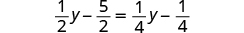Multiply by the LCD, 4.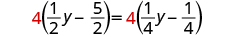Distribute.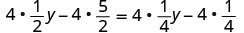Simplify.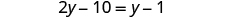Collect the variables to the left.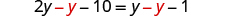Simplify.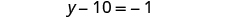Collect the constants to the right.Simplify.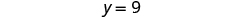Check: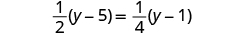Let $y=9$ .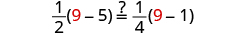Finish the check on your own.

Solve: $\frac{1}{5}\left(n+3\right)=\frac{1}{4}\left(n+2\right)$ .

$n=2$

Solve: $\frac{1}{2}\left(m-3\right)=\frac{1}{4}\left(m-7\right)$ .

$m=-1$

Solve: $\frac{5x-3}{4}=\frac{x}{2}$ .

## Solution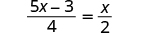Multiply by the LCD, 4.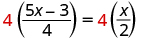Simplify.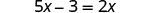Collect the variables to the right.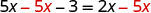Simplify.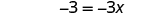Divide.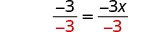Simplify.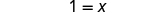Check: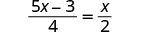Let $x=1$ .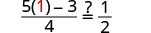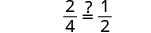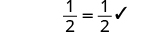Solve: $\frac{4y-7}{3}=\frac{y}{6}$ .

$y=2$

Solve: $\frac{-2z-5}{4}=\frac{z}{8}$ .

$z=-2$

Solve: $\frac{a}{6}+2=\frac{a}{4}+3$ .

## Solution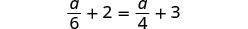Multiply by the LCD, 12.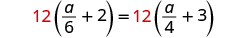Distribute.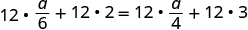Simplify.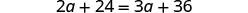Collect the variables to the right.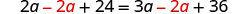Simplify.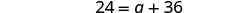Collect the constants to the left.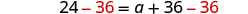Simplify.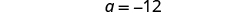Check: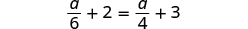Let $a=-12$ .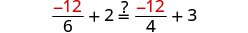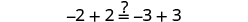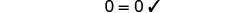Solve: $\frac{b}{10}+2=\frac{b}{4}+5$ .

$b=-20$

Solve: $\frac{c}{6}+3=\frac{c}{3}+4$ .

$c=-6$

Solve: $\frac{4q+3}{2}+6=\frac{3q+5}{4}$ .

## Solution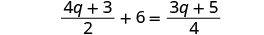Multiply by the LCD, 4.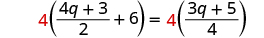Distribute.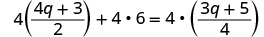Simplify.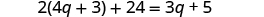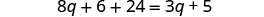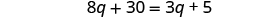Collect the variables to the left.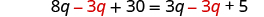Simplify.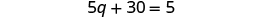Collect the constants to the right.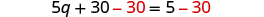Simplify.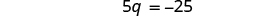Divide by 5.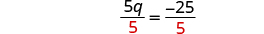Simplify.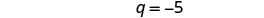Check: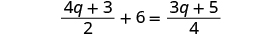Let $q=-5$ .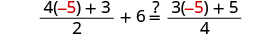Finish the check on your own.

Solve: $\frac{3r+5}{6}+1=\frac{4r+3}{3}$ .

$r=1$

Solve: $\frac{2s+3}{2}+1=\frac{3s+2}{4}$ .

$s=-8$

## Solve equations with decimal coefficients

Some equations have decimals in them. This kind of equation will occur when we solve problems dealing with money or percentages. But decimals can also be expressed as fractions. For example, $0.3=\frac{3}{10}$ and $0.17=\frac{17}{100}$ . So, with an equation with decimals, we can use the same method we used to clear fractions—multiply both sides of the equation by the least common denominator.

Solve: $0.06x+0.02=0.25x-1.5$ .

## Solution

Look at the decimals and think of the equivalent fractions.

$0.06=\frac{6}{100}\phantom{\rule{2em}{0ex}}0.02=\frac{2}{100}\phantom{\rule{2em}{0ex}}0.25=\frac{25}{100}\phantom{\rule{2em}{0ex}}1.5=1\frac{5}{10}$

Notice, the LCD is 100.

By multiplying by the LCD, we will clear the decimals from the equation.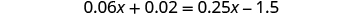Multiply both sides by 100.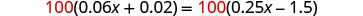Distribute.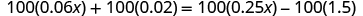Multiply, and now we have no more decimals.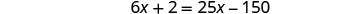Collect the variables to the right.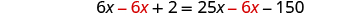Simplify.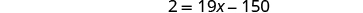Collect the constants to the left.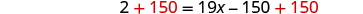Simplify.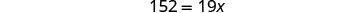Divide by 19.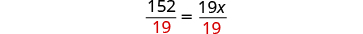Simplify.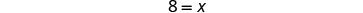Check: Let $x=8$ .Solve: $0.14h+0.12=0.35h-2.4$ .

$h=12$

Solve: $0.65k-0.1=0.4k-0.35$ .

$k=-1$

The next example uses an equation that is typical of the money applications in the next chapter. Notice that we distribute the decimal before we clear all the decimals.

Solve: $0.25x+0.05\left(x+3\right)=2.85$ .

## Solution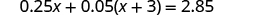Distribute first.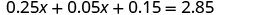Combine like terms.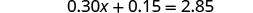To clear decimals, multiply by 100.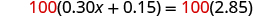Distribute.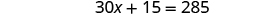Subtract 15 from both sides.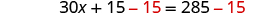Simplify.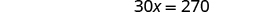Divide by 30.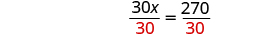Simplify.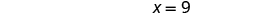Check it yourself by substituting $x=9$ into the original equation.

Solve: $0.25n+0.05\left(n+5\right)=2.95$ .

$n=9$

Solve: $0.10d+0.05\left(d-5\right)=2.15$ .

$d=16$

## Key concepts

• Strategy to Solve an Equation with Fraction Coefficients
1. Find the least common denominator of all the fractions in the equation.
2. Multiply both sides of the equation by that LCD. This clears the fractions.
3. Solve using the General Strategy for Solving Linear Equations.

## Practice makes perfect

Solve Equations with Fraction Coefficients

In the following exercises, solve each equation with fraction coefficients.

$\frac{1}{4}x-\frac{1}{2}=-\frac{3}{4}$

$\frac{3}{4}x-\frac{1}{2}=\frac{1}{4}$

$x=1$

$\frac{5}{6}y-\frac{2}{3}=-\frac{3}{2}$

$\frac{5}{6}y-\frac{1}{3}=-\frac{7}{6}$

$y=-1$

$\frac{1}{2}a+\frac{3}{8}=\frac{3}{4}$

$\frac{5}{8}b+\frac{1}{2}=-\frac{3}{4}$

$b=-2$

$2=\frac{1}{3}x-\frac{1}{2}x+\frac{2}{3}x$

$2=\frac{3}{5}x-\frac{1}{3}x+\frac{2}{5}x$

$x=3$

$\frac{1}{4}m-\frac{4}{5}m+\frac{1}{2}m=-1$

$\frac{5}{6}n-\frac{1}{4}n-\frac{1}{2}n=-2$

$n=-24$

$x+\frac{1}{2}=\frac{2}{3}x-\frac{1}{2}$

$x+\frac{3}{4}=\frac{1}{2}x-\frac{5}{4}$

$x=-4$

$\frac{1}{3}w+\frac{5}{4}=w-\frac{1}{4}$

$\frac{3}{2}z+\frac{1}{3}=z-\frac{2}{3}$

$z=-2$

$\frac{1}{2}x-\frac{1}{4}=\frac{1}{12}x+\frac{1}{6}$

$\frac{1}{2}a-\frac{1}{4}=\frac{1}{6}a+\frac{1}{12}$

$a=1$

$\frac{1}{3}b+\frac{1}{5}=\frac{2}{5}b-\frac{3}{5}$

$\frac{1}{3}x+\frac{2}{5}=\frac{1}{5}x-\frac{2}{5}$

$x=-6$

$1=\frac{1}{6}\left(12x-6\right)$

$1=\frac{1}{5}\left(15x-10\right)$

$x=1$

$\frac{1}{4}\left(p-7\right)=\frac{1}{3}\left(p+5\right)$

$\frac{1}{5}\left(q+3\right)=\frac{1}{2}\left(q-3\right)$

$q=7$

$\frac{1}{2}\left(x+4\right)=\frac{3}{4}$

$\frac{1}{3}\left(x+5\right)=\frac{5}{6}$

$x=-\frac{5}{2}$

$\frac{5q-8}{5}=\frac{2q}{10}$

$\frac{4m+2}{6}=\frac{m}{3}$

$m=-1$

$\frac{4n+8}{4}=\frac{n}{3}$

$\frac{3p+6}{3}=\frac{p}{2}$

$p=-4$

$\frac{u}{3}-4=\frac{u}{2}-3$

$\frac{v}{10}+1=\frac{v}{4}-2$

$v=20$

$\frac{c}{15}+1=\frac{c}{10}-1$

$\frac{d}{6}+3=\frac{d}{8}+2$

$d=-24$

$\frac{3x+4}{2}+1=\frac{5x+10}{8}$

$\frac{10y-2}{3}+3=\frac{10y+1}{9}$

$y=-1$

$\frac{7u-1}{4}-1=\frac{4u+8}{5}$

$\frac{3v-6}{2}+5=\frac{11v-4}{5}$

$v=4$

Solve Equations with Decimal Coefficients

In the following exercises, solve each equation with decimal coefficients.

$0.6y+3=9$

$0.4y-4=2$

$y=15$

$3.6j-2=5.2$

$2.1k+3=7.2$

$k=2$

$0.4x+0.6=0.5x-1.2$

$0.7x+0.4=0.6x+2.4$

$x=20$

$0.23x+1.47=0.37x-1.05$

$0.48x+1.56=0.58x-0.64$

$x=22$

$0.9x-1.25=0.75x+1.75$

$1.2x-0.91=0.8x+2.29$

$x=8$

$0.05n+0.10\left(n+8\right)=2.15$

$0.05n+0.10\left(n+7\right)=3.55$

$n=19$

$0.10d+0.25\left(d+5\right)=4.05$

$0.10d+0.25\left(d+7\right)=5.25$

$d=10$

$0.05\left(q-5\right)+0.25q=3.05$

$0.05\left(q-8\right)+0.25q=4.10$

$q=15$

## Everyday math

Coins Taylor has $2.00 in dimes and pennies. The number of pennies is 2 more than the number of dimes. Solve the equation $0.10d+0.01\left(d+2\right)=2$ for $d$ , the number of dimes. Stamps Paula bought$22.82 worth of 49-cent stamps and 21-cent stamps. The number of 21-cent stamps was 8 less than the number of 49-cent stamps. Solve the equation $0.49s+0.21\left(s-8\right)=22.82$ for s , to find the number of 49-cent stamps Paula bought.

$s=35$

## Writing exercises

Explain how you find the least common denominator of $\frac{3}{8}$ , $\frac{1}{6}$ , and $\frac{2}{3}$ .

If an equation has several fractions, how does multiplying both sides by the LCD make it easier to solve?

If an equation has fractions only on one side, why do you have to multiply both sides of the equation by the LCD?

In the equation $0.35x+2.1=3.85$ what is the LCD? How do you know?

100. Justifications will vary.

## Self check

After completing the exercises, use this checklist to evaluate your mastery of the objectives of this section.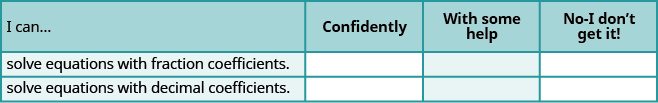Overall, after looking at the checklist, do you think you are well-prepared for the next section? Why or why not?

Nga and Lauren bought a chest at a flea market for $50. They re-finished it and then added a 350 % mark - up Makaila Reply the sum of two Numbers is 19 and their difference is 15 Abdulai Reply 2, 17 Jose interesting saw Felecia left her home to visit her daughter, driving 45mph. Her husband waited for the dog sitter to arrive and left home 20 minutes, or 13 hour later. He drove 55mph to catch up to Felecia. How long before he reaches her? Rafi Reply integer greater than 2 and less than 12 Emily Reply 2 < x < 12 Felix I'm guessing you are doing inequalities... Felix Actually, translating words into algebraic expressions / equations... Felix hi Darianna hello Mister Eric here Eric He charges$125 per job. His monthly expenses are $1,600. How many jobs must he work in order to make a profit of at least$2,400?
at least 20
Ayla
what are the steps?
Alicia
6.4 jobs
Grahame
32
Grahame
1600+2400= total amount with expenses. 4000/125= number of jobs needed to make that min profit of 2400. answer is 32
Orlando
He must work 32 jobs to make a profit
POP
what is algebra
repeated addition and subtraction of the order of operations. i love algebra I'm obsessed.
Shemiah
hi
Krekar
Eric here. I'm a parent. 53 years old. I have never taken algebra. I want to learn.
Eric
One-fourth of the candies in a bag of M&M’s are red. If there are 23 red candies, how many candies are in the bag?
they are 92 candies in the bag
POP
rectangular field solutions
What is this?
Donna
t
muqtaar
the proudact of 3x^3-5×^2+3 and 2x^2+5x-4 in z7[x]/ is
?
Choli
a rock is thrown directly upward with an initial velocity of 96feet per second from a cliff 190 feet above a beach. The hight of tha rock above the beach after t second is given by the equation h=_16t^2+96t+190
Usman
Stella bought a dinette set on sale for $725. The original price was$1,299. To the nearest tenth of a percent, what was the rate of discount?
44.19%
Scott
40.22%
Terence
44.2%
Orlando
I don't know
Donna
if you want the discounted price subtract $725 from$1299. then divide the answer by $1299. you get 0.4419... but as percent you get 44.19... but to the nearest tenth... round .19 to .2 and you get 44.2% Orlando you could also just divide$725/$1299 and then subtract it from 1. then you get the same answer. Orlando p mulripied-5 and add 30 to it Tausif Reply p mulripied-5 and add30 Tausif p mulripied-5 and addto30 Tausif how muqtaar Can you explain further Monica Reply p mulripied-5 and add to 30 Tausif -5p+30? Corey p=-5+30 Jacob How do you find divisible numbers without a calculator? Jacob Reply TAKE OFF THE LAST DIGIT AND MULTIPLY IT 9. SUBTRACT IT THE DIGITS YOU HAVE LEFT. IF THE ANSWER DIVIDES BY 13(OR IS ZERO), THEN YOUR ORIGINAL NUMBER WILL ALSO DIVIDE BY 13!IS DIVISIBLE BY 13 BAINAMA When she graduates college, Linda will owe$43,000 in student loans. The interest rate on the federal loans is 4.5% and the rate on the private bank loans is 2%. The total interest she owes for one year was \$1,585. What is the amount of each loan?
Sean took the bus from Seattle to Boise, a distance of 506 miles. If the trip took 7 2/3 hours, what was the speed of the bus?
66miles/hour
snigdha
How did you work it out?
Esther
s=mi/hr 2/3~0.67 s=506mi/7.67hr = ~66 mi/hr
Orlando
No; 65m/hr
albertBy Brooke DelaneyBy OpenStaxByBy Angelica LitoBy Richley CrapoBy OpenStaxBy OpenStaxBy OpenStaxBy OpenStaxBy Ryan Lowe